# 前言

看完后也是久久不能平静，以至于我开始翻箱倒柜地找我之前写的那些物理学笔记和论文(关于光学和量子物理的)，准备继续下去，然而没有找到，我很焦急，我想到它们可能在老家，也可能在上海的家里，我甚至都想抽个周末飞回上海去寻找这些东西，这想法有点疯狂，但这就是事实。

老婆带着小小去北京参加比赛了，留我自己在家，临走还帮我塞满了一冰箱啤酒，真是体贴…今天周末，没有工作的遗留，没有家务事，我想是时候写点关于《三体》的一点思考了。
把思绪整理成文章是一件比较困难的事情，有时终于写完了，却发现文中并没有包括全部的思路，于是就是补遗再补遗，这比写那种技术总结难多了，真是的。不管怎样，我想试试看，如果有遗漏的东西，只好后面再补遗和润色了。

# 正文

在整体构思和框架布局上，我觉得《三体》是非常优秀的，虽然大刘的文笔在叙事上稍显拖沓，但也展现了几个个性鲜明的人物形象，也还算过关，当然，这方面是远远没法和《盗墓》系列比的。不过本文不准备扯这些，这些今后会专门写一篇文章，本文是想解释三个概念，即画像，降维打击以及黑暗森林，其中牵扯的东西会比较多比较杂，也不一定都是对的，我想说这篇文章只是记录了一个思考的过程，它并不是学术论文或者技术文档，算是一篇随笔吧。

## 1.画像与泰勒展开

画师作画和工程师不同，画师不会去测量，只是去感悟，去观察就好了，然后把大脑里加工后的东西用线条展现出来。
在古希腊，曾经有一个毕达哥拉斯学派，在他们的眼里，宇宙就是数与数的关系构成的，数是组成宇宙的基本元素，他们试图用各种方法去直观地用数表示各种关系，从而发明了超级多的“具有几何意义”的算术，然而有一天，一位叫做希帕索斯的门徒，发现了一个不可名状的关系，导致了整个哲学体系面临崩塌的危险，这个希帕索斯也为惹这么大的事付出了生命的代价，现在我们知道，这个希帕索斯所谓的不可名状的关系就是2$\sqrt{2}$.现在我们还知道，数学界早就可以为毕达哥拉斯学派眼里“不可名状的关系”来“画像”了。

自然对数e$e$是个什么东西，如何去描述它，它也是不可名状的，就像2$\sqrt{2}$，π一样…还记得云天明讲给程心的那三个故事吗？针眼画师画像的故事，如果给他一个e$e$，他要怎么把它画出来？
幸运的是，在我们这个真实的世界，就曾经有一位针眼画师，布鲁克.泰勒，他向e$e$扔去了一块二向箔，于是e$e$就被他刻画出来了。
我们来猜测一下，他是怎么做到的。
通过阅读《三体》小说以及学过的一些数学知识，加上我自己的一些思考，我总结出了两点原则：

1.自然界是全息的，分形的，从一个点可以窥见整个世界；
2.自然界是多维的，通过不断的降维，我们就可以完全认识它。

首先，我们先来定义一下什么是简单的，什么是复杂的不可名状的。简单点说，像axn+bxm+cxp...π$ax^n+bx^m+cx^p...这种二项式就是简单的，因为它是可是手算的，完全靠四则混合运算就能搞得定，那些相反，像π，$e$，正弦函数，余弦函数等三角函数这些就是复杂的，如果我问$sin(20)\$等于几，你要么查表，要么用计算器，反正用四则混合运算你是搞不定的…
如果能用二项式的组合去拟合任意曲线，那么这种组合的方法将会成为画师最强有力的画笔，而这是完全可能的，我们只需要一些可以映射到坐标系y轴任意位置的二项式函数，用这些函数组合起来就可以得到任意函数的拟合函数。我有一个示意图如下：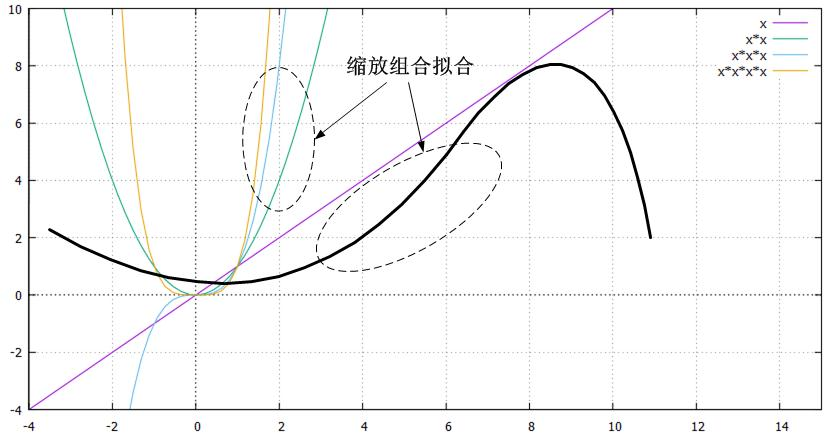## f(x)=sin(x)f(x)=sin(x)

我试图在本文中通过sinx来解释。我们画出sinx的图像如下：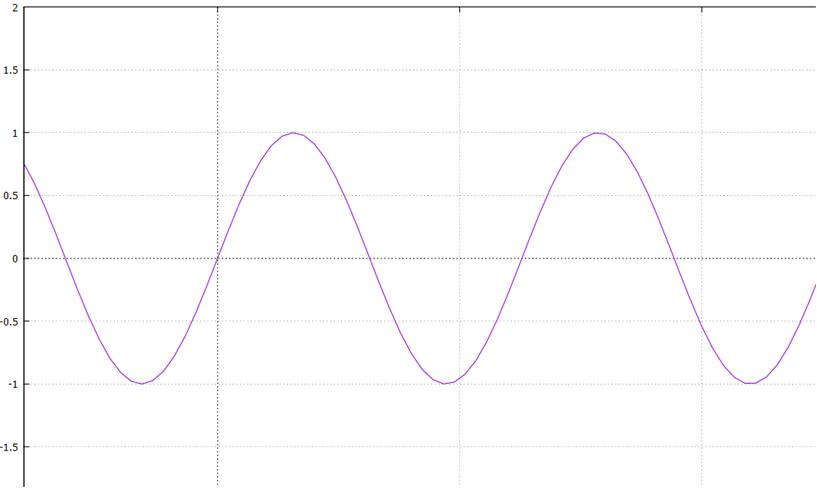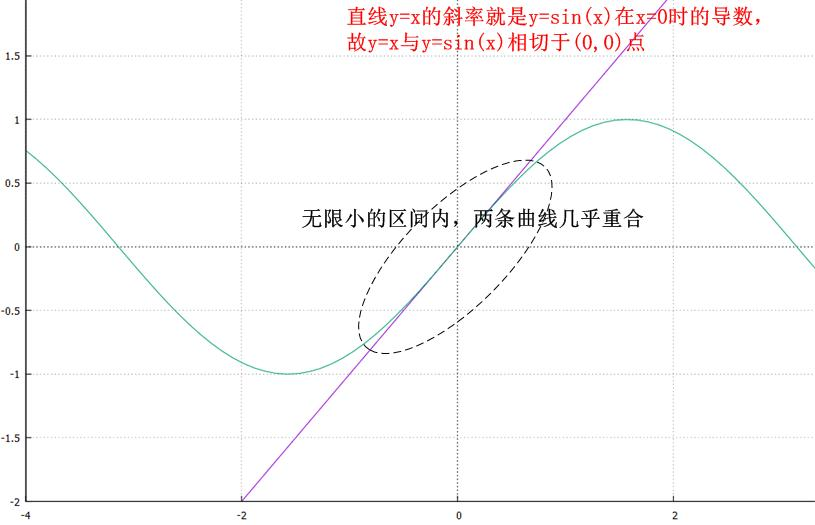暂且打住，经过原点的直线多了，随便举一个例子，y=3x$y=3x$，这条直线上经过原点的点坐标也是(0,0)$(0,0)$，它和y=sin(x)$y=sin(x)$(0,0)$(0,0)$是不是也相同呢？答案是完全不同！当我们说两条曲线上对应的两个点相同的时候，有两层含义：

• 两个点的坐标相同；
• 两个点的走势相同。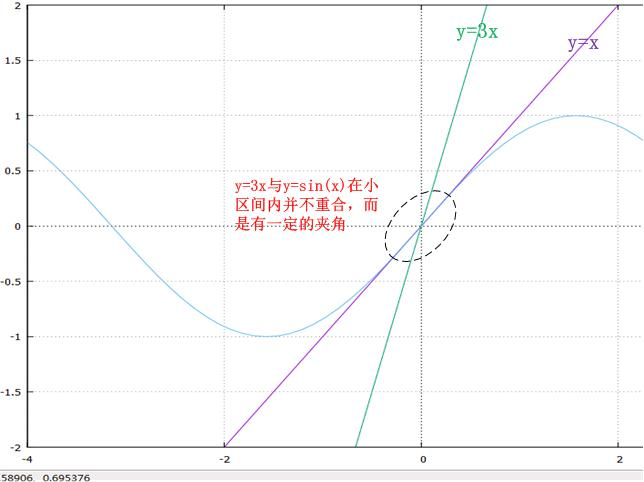## limx→0sin(x)3x=13 \lim_{x \to 0}\frac{sin(x)}{3x}=\frac{1}{3}

答案就是切割，降维！当然，在数学上就是不断求导，其实我不喜欢“求导”这种说法。我们需要用切割，降维，或者说求导(以后就说求导吧，这样更专业些)方法，把蕴含在这单独的一个点中的信息给拽出来，我们现在在做的事情就是从一颗受精卵里拽出一个成人，从一颗粒子中观看整个世界。
对一个函数求导可以得到另一个函数，该点的坐标代入这另一个函数所得到的函数值表示原函数在该点的走势，这个走势包含的意思包括两个方面，曲线延展的强度大小和曲线延展方向，换句话说，它是个矢量。有了这个走势信息，我们就能预测在稍微偏理原点时，函数曲线的大致走向了，这就使得对整个y=sin(x)$y=sin(x)$的画像向前又描了一笔。事实上，y=x$y=x$这条直线就是y=sin(x)$y=sin(x)$在原点处的切线，它可以在原点附近近似代替y=sin(x)$y=sin(x)$，换句话说这条直线的斜率1是对y=sin(x)$y=sin(x)$求导得到导函数y=cos(x)$y=cos(x)$x=0$x=0$时的y值，它预示着y=sin(x)$y=sin(x)$的走势就是从原点开始仰角45°斜向上，除此之外就不再有任何信息了.
既然我们知道了这个信息，那么我们就可以通过导函数y=cos(x)$y=cos(x)$再次求导，得到原函数y=sin(x)$y=sin(x)$走势的走势，而这个走势的走势在离开原点再远一点的x点处体现，很遗憾，我们选的原点代入导函数的导函数y=sin(x)$y=-sin(x)$后结果为0，不过没关系，继续就是了，再对它求导，导函数是y=cos(x)$y=-cos(x)$，x=1代入，得到-1，我们得到了y=sin(x)$y=sin(x)$在x=0处的“走势的走势的走势”，下面看下效果：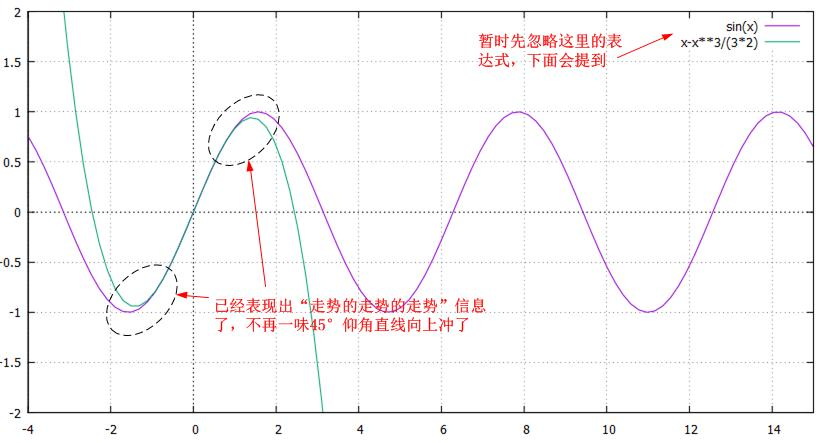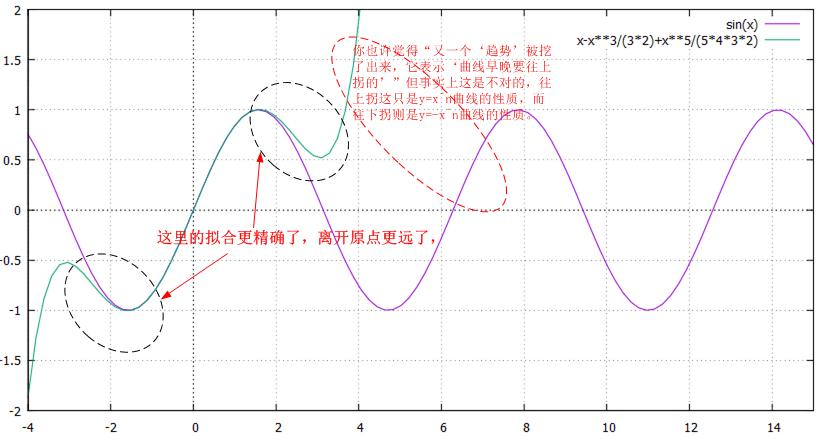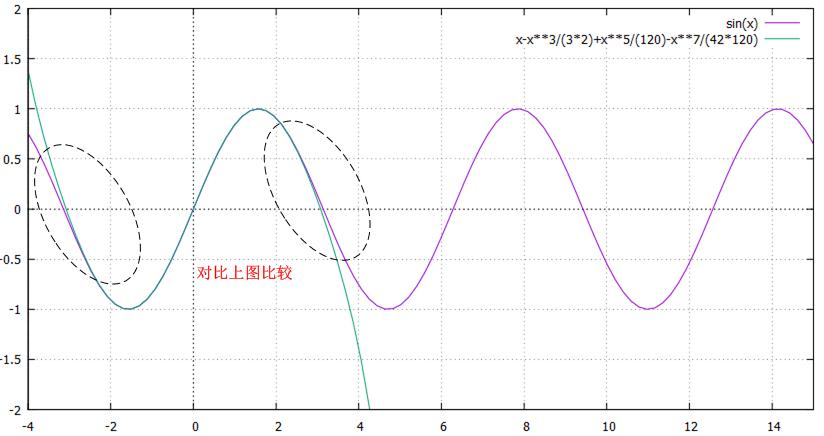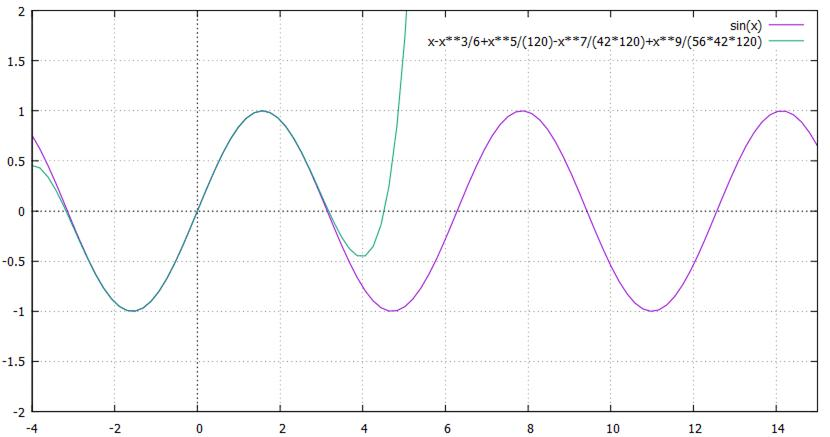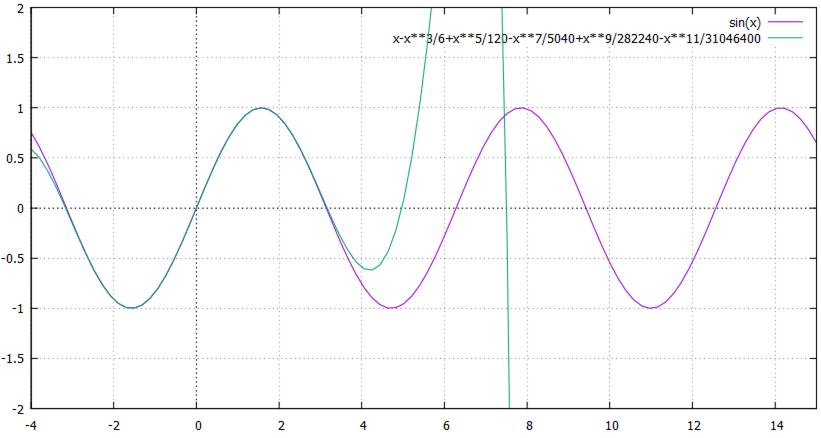还记得我们的宗旨吗？用二项式函数曲线去拟合任意函数曲线，也许你会问当最终的表达式写出来前，是如何确定每一个二项式项的系数的，其实这个并不是理论的重点，而纯粹是一个“技术”活儿，完全可以通过待定系数法确定系数，以下是一个简单的推导过程，并不严谨，但可以说明问题：

f(x)=f(p)+a1(xp)+a2(xp)2+a3(xp)3...$f(x)=f(p)+a_1(x-p)+a_2(x-p)^2+a_3(x-p)^3...$

f(1)(x)=0+a1+2a2(xp)+3a3(xp)2...$f^{(1)}(x) = 0+a_1+2a_2(x-p)+3a_3(x-p)^2...$
f(2)(x)=0+0+2a2+3!a3(xp)+...$f^{(2)}(x) = 0+0+2a_2+3!a_3(x-p)+...$
f(3)(x)=0+0+0+3!a3+...$f^{(3)}(x) = 0+0+0+3!a_3+...$

f(1)(p)=1!a1$f^{(1)}(p) = 1!a_1$
f(2)(p)=2!a2$f^{(2)}(p) = 2!a_2$
f(3)(p)=3!a3$f^{(3)}(p) = 3!a_3$

a1=f(1)(p)1!$a_1=\frac{f^{(1)}(p)}{1!}$
a2=f(2)(p)2!$a_2=\frac{f^{(2)}(p)}{2!}$
a3=f(3)(p)3!$a_3=\frac{f^{(3)}(p)}{3!}$

f(x)=f(p)+f(1)(p)1!(xp)+f(2)(p)2!(xp)2+f(3)(p)3!(xp)3...$f(x)=f(p)+\frac{f^{(1)}(p)}{1!}(x-p)+\frac{f^{(2)}(p)}{2!}(x-p)^2+\frac{f^{(3)}(p)}{3!}(x-p)^3...$

多阶导数的不断求解是一个不断修正的过程，画过素描的应该都知道这个过程是怎么进行的。
好吧，到此为止，应该把这个说清楚了，并且我们已经知道了y=sin(x)$y=sin(x)$到底长什么样子了，这一切就是布鲁克.泰勒的泰勒级数所要展示的画卷。
泰勒发现了自然界的秘密，从一个点看世界，如果我们把世界的元素在时间轴上拉伸，就可以看出，每一个元素，不管再渺小都是沿着时间轴按照自己的内在趋势发展的，因此当我们把所谓的趋势也维度化的时候，就会发现，每一维度的趋势组成了一个正交基，在数学上，这正是通过一个函数表达式的各阶导数来展现的，通俗点说，这个正交基就是：

(原值，趋势，趋势的趋势，趋势的趋势的趋势，趋势的趋势的趋势的趋势，趋势的趋势的趋势的趋势的趋势，…)

除了泰勒展开，还有更好玩的，那就是傅立叶展开。
如果说泰勒展开是把任意曲线用“各阶趋势”的分量求和表示的话，那么傅立叶展开则试图将任意曲线表示成“波形的叠加”。跟主音吉他手讨论这个问题，主音说，一个音就是无数个音。
和泰勒的思想一样，一就是所有。
泰勒展开和傅立叶展开，区别是什么？按照不同的规则作画，规则不同而已，国画和油画的区别？随便怎么说吧，反正就是这个意思。

## 2.歌者的降维打击

我们高中有一个走路步伐比较猛的同学，比较能打架，后来做了刑警，他打架的时候一般不是气冲冲地打，而是唱着歌，微笑着，时而嘴里夹着咒骂，一般摇摆身躯一遍打，但是跟尼古拉斯赵四不同，他唱歌摇摆的时候已经开打了…歌者正是这样的风格。这部小说中，我喜欢的人不多，大致就是叶文洁，史强，章北海，歌者，AA，智子这六人，如果你也看过小说，就会发现，这六人有个共同点，那就是做事理智，不含糊，几乎从不煽情，几乎从不动容，和汪淼，逻辑，程心，云天明，关一帆，三体联络员这些事儿精的矫情之人完全不同。但在这六人中，只有歌者是一边感慨一边工作，而且感慨丝毫不耽误工作。

名称不重要，现在的问题是，就像逻辑，程心他们在冥王星保存地球博物馆遗迹时所期望的那样，已经二维化的太阳系真的可以在以后被还原吗？
首先，我们看一下蓝色空间号在四维干掉水滴的事，没看过小说的只要了解到一个事实就好了，在高一维的空间中，可以肆意突破低维的任意障碍。我以下图举例：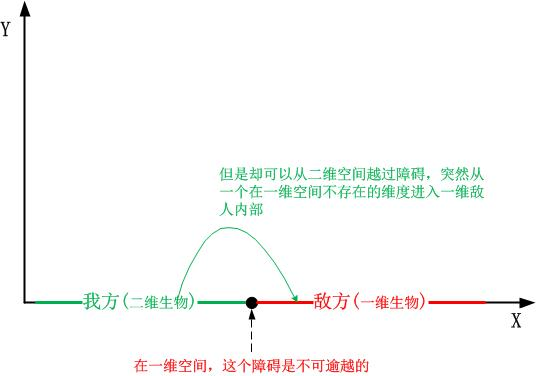这只是事情的一个方面，事情的另一方面是，所谓的降维并不是简单的垂直映射和投影，因为这必然会丢失一个维度的信息，然而信息不可能凭空丢失，它是守恒的。另外，降维打击也不是一个“踩扁”的过程，因为就算踩的再扁，也还是会有厚度的，毕竟再剧烈的操作都是在实施攻击的维度内进行的，在同一维度，不可能凭空让一个维度消失，因此，降维打击实际上是一个重新映射的过程，比如可以把二维平面的点(x,y)$(x,y)$映射成一维平面的方程组：

{ax=c1by=c2

• 1.适应–掌握映射规则，自己在降维后的空间里继续生存；
• 2.改变–掌握积分规则，它求导你就积分，与之对抗；
• 3.随缘–把自己变成y=ex$y=e^x$，金刚不坏（y=ex$y=e^x$导数是自身）.

这里我曾经有一个疑问，那就是，即便地球文明掌握了二维化的映射规则，人体能挡得住那种强度的物理攻击吗？就像人被吸入黑洞或者进入虫洞到达另外一个地方一样，在那一瞬间，人的肉身就会解体，即便残骸到了另一个世界又能如何？因此即便掌握了映射规则，如果不做前置工作，个体的死亡依然是不可避免的。能存活的可能只是地球文明的人类按照逆二维化规则保存下来的“自己文明的证明”吧。
我们已经看到了从低维到高维的意义以及从高维到低维的映射，那么问题是，一个低维的物体能顺利在高维空间存在吗？以我们三维空间为例，我们的物理规律比如距离的平方反比律，关于体积的立方律，为什么是平方，为什么是立方，这些都是跟空间维度有关的，如果到了四维空间，距离岂不成了立方反比律了？再考虑构成我们世界的基本粒子，无一不是遵循着这些与空间维度有关的各种物理规律，一旦到达高维空间或者低维空间而不做任何前置工作的话，基本粒子之间的作用力规律会完全疯掉吧…基本粒子都疯了，一个卑微的凡人如何保持体面？
(TODO)

## 3.黑暗森林和猜疑链

职场就是个黑暗森林，不管同事之间的关系再好，如果可能，谁都想不由分说，灭了对方，因为资源总量是一定的，每个人都要在职业道路上往上走，除非，除非你发表安全声明。然而通过第三部《死神永生》我们知道，所谓的安全声明就是禁锢自己，而这在职场上不可能的。
理解了这个，我便觉得大多数公司的薪资保密制度确实是多此一举了，按照黑暗森林法则(前提你得相信它)，每个人最安全的做法就是隐藏自己，隐瞒自己的一切，长者不也是很赞同“闷声发大财”这一说吗？有谁会跟别人透露自己真实的薪资？
如果对方告诉你他可以拿到2万，而此时你只有1.5万的时候，你会相信他说的吗？你告诉他你只有1.5万的时候，他会相信你吗？你怎么知道他是怎么想你的，万一他相信了，他又是怎么揣测你的怎么想他的，….这个链条会一直递归到你的大脑栈溢出，这是一种没有意义的能量损耗，所以说按照收益最大化的原则，要么隐瞒，要么先下手为强。
其实，黑暗森林的猜疑链只是整个黑暗森林的一个局部，优胜劣汰，弱肉强食，这是亘古不变的真理，与之相对应，便出现了各种应对措施，比如法，术，势，阴谋，阳谋，王道，霸道，厚黑…不管采用什么措施，最终记住一点就好，那就是马太效应，来自《新约·马太福音》：

## 4.还有一些没说的

我尽量不把那些复杂的东西包含在文中，比如线性代数，秩，偏振光…我记起，当时的几大本子的笔记绝大部分都是写量子力学，偏振光的，如果今天写那些，估计这个周末要完全泡汤，又有极大的可能性会影响工作日的工作，所以只能打住，我也对自己感到抱歉，其实闭上眼睛，我是多么快乐地回忆起那时高中，大学的两个教物理的刘老师，历历在目，恍若昨日！

# 后记

©️2019 CSDN 皮肤主题: 编程工作室 设计师: CSDN官方博客